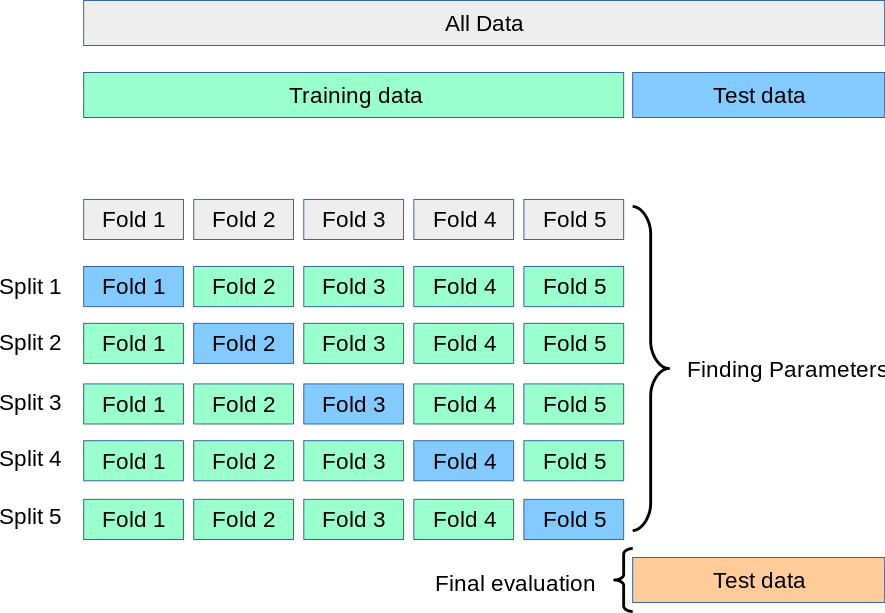# K-Fold Cross Validation using Scikit-Learn in Python

Learn how you can perform K-Fold cross validation technique using the scikit-learn library in Python.
5 min read · Updated may 2022 · Machine Learning

When training data in supervised machine learning, We're not looking for how well the model performs on the training data but on new data (e.g., a new customer, a new crime, a new image, etc.). Consequently, our approach to assessment should allow us to examine how effectively models can predict from data they have never seen before.

You may want to hold back a portion of the data to test a hypothesis. We term this process "validation" (or hold-out). Observation (features and targets) are divided into two sets during validation, known as the training and test sets.

We set the test set aside to maintain the illusion that we've never seen it. In the next step, we use training data to teach our model how to generate the most accurate predictions. As a final step, we evaluate the performance of our model that is trained on our training set on our test set.

There are some flaws in this strategy. There will not be enough data in training set for the model to learn the relationship between the inputs and the outputs for small datasets, and the k-fold cross-validation procedure will be suitable here.

K-fold cross-validation (KFCV) is a technique that divides the data into `k` pieces termed "folds". The model is then trained using `k - 1` folds, which are integrated into a single training set, and the final fold is used as a test set. This is repeated `k` times, each time using a different fold as the test set. The model's performance is then averaged across the `k` iterations to provide an overall measurement.source: scikit-learn.org

For the demo of this tutorial, make sure you have the scikit-learn package installed:

``\$ pip install scikit-learn``

``````# Load libraries
from sklearn import datasets
from sklearn import metrics
from sklearn.model_selection import KFold, cross_val_score
from sklearn.pipeline import make_pipeline
from sklearn.linear_model import LogisticRegression
from sklearn.preprocessing import StandardScaler``````

We'll be using the simple digits dataset as a demonstration for this tutorial:

``````# digits dataset loading
# Create features matrix
features = digits.data
# Create target vector
target = digits.target``````

Making our `StandardScaler` and the model, in this case, we'll choose `LogisticRegression()`:

``````# standardization
standard_scaler = StandardScaler()
# logistic regression creation
logit = LogisticRegression()``````

To get the KFold cross-validation score in Scikit-learn, you can use `KFold` class and pass it to the `cross_val_score()` function, along with the pipeline (preprocessing and model) and the dataset:

``````# pipeline creation for standardization and performing logistic regression
pipeline = make_pipeline(standard_scaler, logit)
# perform k-Fold cross-validation
kf = KFold(n_splits=11, shuffle=True, random_state=2)
# k-fold cross-validation conduction
cv_results = cross_val_score(pipeline, # Pipeline
features, # Feature matrix
target, # Target vector
cv=kf, # Cross-validation technique
scoring="accuracy", # Loss function
n_jobs=-1) # Use all CPU cores
# View score for all 11 folds
cv_results``````
``````array([0.92682927, 0.98170732, 0.95731707, 0.95121951, 0.98159509,
0.97546012, 0.98159509, 0.98773006, 0.96319018, 0.97546012,
0.96932515])``````

We used k-fold cross-validation with 11 folds in our solution. Let's calculate the mean CV score:

``````# Calculate mean
cv_results.mean()``````
``0.968311727177506``

The `cross_val_score()` function accepts several parameters:

• `estimator`: The object to use to fit the data, either an estimator or a pipeline like in our case.
• `X` and `y`: The dataset inputs and outputs.
• Our cross-validation approach is determined by the `cv` parameter. K-fold is by far the most popular, although there are others, such as leave-one-out cross-validation, in which the number of folds `k` matches the number of observations.
• The `scoring` parameter defines our success measure.
• Finally, `n_jobs=-1` instructs scikit-learn to employ every available core. For example, if your computer has four cores (which is common in laptops), scikit-learn will utilize all four cores simultaneously to speed up the procedure.

When you perform KFCV, there are three things you need to keep in mind:

• Since each observation is presumed to have been independently generated, KFCV begins with the premise that the data is independent and identically distributed (IID). IID datasets should be shuffled when assigned to folds. Set `shuffle=True` in the `KFold` class to conduct shuffling.
• Second, when using KFCV to assess a classifier, it is frequently valuable to have folds that contain nearly the same proportion of data from each target class (stratified k-fold). If we want to perform stratified KFold cross-validation in scikit-learn, we can simply replace the `KFold` class with `StratifiedKFold`.
• When employing validation sets or cross-validation, it is crucial to preprocess data based on the training set and then apply those transformations to both the training and test sets. The reason for this is that we are claiming that the test data is unknown data. When we fit both of our preprocessors using observations from the training and test sets, some information from the test set seeps into our training set. This rule holds true for every preprocessing step, including feature selection. The pipeline module in scikit-learn makes this simple while using cross-validation approaches. We start by building a pipeline that preprocesses the data (e.g., `standard_scaler`) before training a model (logistic regression, logit): for every preprocessing step, including feature selection.
• KFCV is the preferred method for smaller datasets over train/test splits. However, it is computationally expensive, especially when the chosen `k` is high.

Note: You may configure cross-validation such that the fold size is one (k is set to the number of observations in your dataset). As discussed in the tutorial, this kind of cross-validation is known as leave-one-out cross-validation. Consequently, many performance indicators may be aggregated to assess your model's accuracy on unseen data properly. One disadvantage is that it might be a more computationally costly technique than k-fold cross-validation.

Get the complete demo code here.

Learn also: Dropout Regularization using PyTorch in Python.

Happy learning ♥

Sharing is caring!

### Comment panel